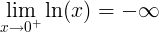# Natural Logarithm Rules & Properties

Rule name Rule Example
##### Product rule

ln(x ∙ y) = ln(x) + ln(y)

ln(37) = ln(3) + ln(7)

##### Quotient rule

ln(x / y) = ln(x) - ln(y)

ln(3 / 7) = ln(3) - ln(7)

##### Power rule

ln(x y) = y ∙ ln(x)

ln(28) = 8ln(2)

##### Ln derivative

f (x) = ln(x) f ' (x) = 1 / x

##### Ln integral

ln(x)dx = x ∙ (ln(x) - 1) + C

##### Ln of negative number

ln(x) is undefined when x ≤ 0

##### Ln of zero

ln(0) is undefinedln(1) = 0

##### Ln of infinity

lim ln(x) = ∞ , when x→∞

#### Derivative of natural logarithm (ln) function

The derivative of the natural logarithm function is the reciprocal function.

When

f (x) = ln(x)

The derivative of f(x) is:

f ' (x) = 1 / x

#### Integral of natural logarithm (ln) function

The integral of the natural logarithm function is given by:

When

f (x) = ln(x)

The integral of f(x) is:

f (x)dx = ∫ ln(x)dx = x ∙ (ln(x) - 1) + C

Natural logarithm calculator ►